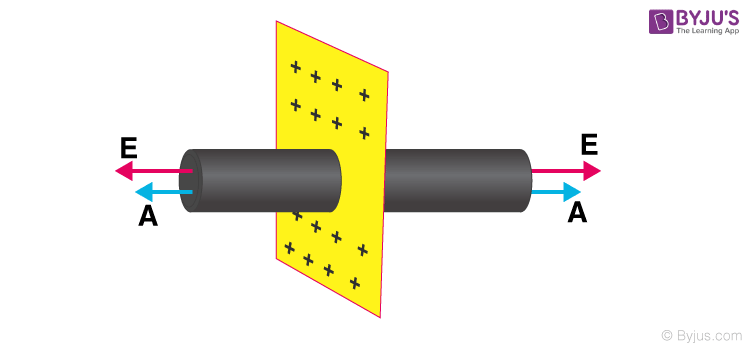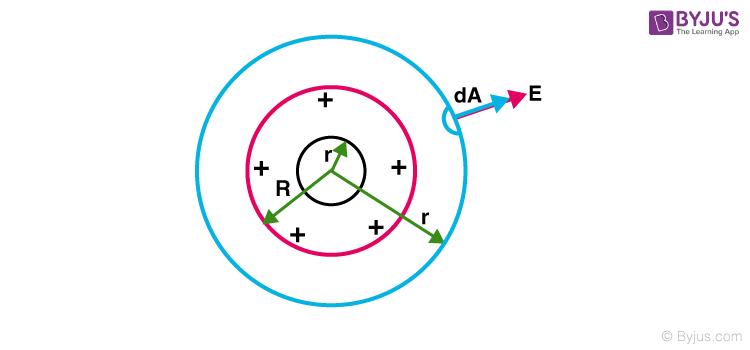# Electric Field Of A Sphere

## Electric Field of an Infinite Plane

Let the surface charge density be σ. In this case, there is planar symmetry and the electric field lies perpendicular to the plane of charge. For a positively charged plane, the field points away from the plane of charge.

We use a cylindrical Gaussian surface that pierces the plane and has its straight faces parallel to the plane. Since the electric field is perpendicular to the plane of charge, it contributes zero flux on the cylinder’s curved surface (θ = 90⁰). The angle between the straight surfaces’ area vector and the electric field is zero.

Let the surface area of the cylinder’s straight face be A as shown in the diagram:Using  Gauss Law,

Φ = ∮

$$\begin{array}{l} \overrightarrow{E}\end{array}$$
.
$$\begin{array}{l} d\overrightarrow{A}\end{array}$$
=
$$\begin{array}{l} \frac {q_{enc}}{\epsilon_0}\end{array}$$

Since there are two surfaces with a finite flux (the straight surfaces of the cylinder; the curved surface contributes to no flux)

Φ = EA + EA =

$$\begin{array}{l} \frac {\sigma~A}{\epsilon_0} \end{array}$$

2EA =

$$\begin{array}{l} \frac {\sigma~A}{\epsilon_0} \end{array}$$

E =

$$\begin{array}{l} \frac {\sigma}{2\epsilon_0}\end{array}$$

Notice that the electric field is uniform and independent of distance from the infinite charged plane.

## Electric field of a sphereConsider a charged spherical shell with a surface charge density σ and radius R. Consider a spherical Gaussian surface with any arbitrary radius r, centered with the spherical shell. By symmetry, the electric field must point radially. Thus, for a Gaussian surface outside the sphere, the angle between electric field and area vector is 0 (cosθ = 1). For a Gaussian surface outside the sphere, the angle between electric field and area vector is 180⁰ (cosθ = -1). We shall consider two cases:

For r>R,

Using Gauss law,

Φ = ∮

$$\begin{array}{l} \overrightarrow{E}.d \overrightarrow{ A}\end{array}$$
=
$$\begin{array}{l} \frac {q_{enc}}{\epsilon_0}\end{array}$$

Φ = ∮ EdA cos 0 =

$$\begin{array}{l} \frac {\sigma.4\pi R^2}{\epsilon_0}\end{array}$$

E ∮ dA =

$$\begin{array}{l} \frac {\sigma.4\pi R^2}{\\epsilon_0}\end{array}$$

$$\begin{array}{l} E.4πr^2 \end{array}$$
=
$$\begin{array}{l} \frac {\sigma.4\pi R^2}{\\epsilon_0}\end{array}$$

E =

$$\begin{array}{l} \frac {\sigma}{\epsilon_0}~\frac{R^2}{r^2}\end{array}$$

Notice that the total charge on the sphere is

$$\begin{array}{l} q_{tot} \end{array}$$
=
$$\begin{array}{l} σ.4πR^2 \end{array}$$

Thus we can also write the above equation as:

E =

$$\begin{array}{l} \frac {1}{4\pi\epsilon_0}~\frac{q_{tot}}{r^2}\end{array}$$

Notice that this is similar to the electric field due to a point charge. Thus outside the sphere, the electric field behaves as though it is due to a point charge (carrying all the charge of the shell) at the centre of the shell.

For r<R,

Consider the black Gaussian surface as shown in the figure. The net charge enclosed is zero. Thus using Gauss law,

Φ = ∮

$$\begin{array}{l} \overrightarrow{E}.d\overrightarrow{A}\end{array}$$
=
$$\begin{array}{l} \frac {q_{enc}}{ε_0}\end{array}$$

∮EdA cos 180 =

$$\begin{array}{l} \frac {q_{enc}}{ε_0}\end{array}$$

$$\begin{array}{l} -E.4πr^2 \end{array}$$
$$\begin{array}{l} \frac {q_{enc}}{ε_0}\end{array}$$

$$\begin{array}{l} -E.4πr^2 \end{array}$$
$$\begin{array}{l} \frac{0}{ε_0}\end{array}$$

E=0

Thus, there is no electric field inside a charged electric shell!

#### Recommended VideoTo learn and practice more on the electric field of a sphere, and for more information on various physics concepts, download BYJU’S – The Learning App.

Test Your Knowledge On Charged Plane Sphere!